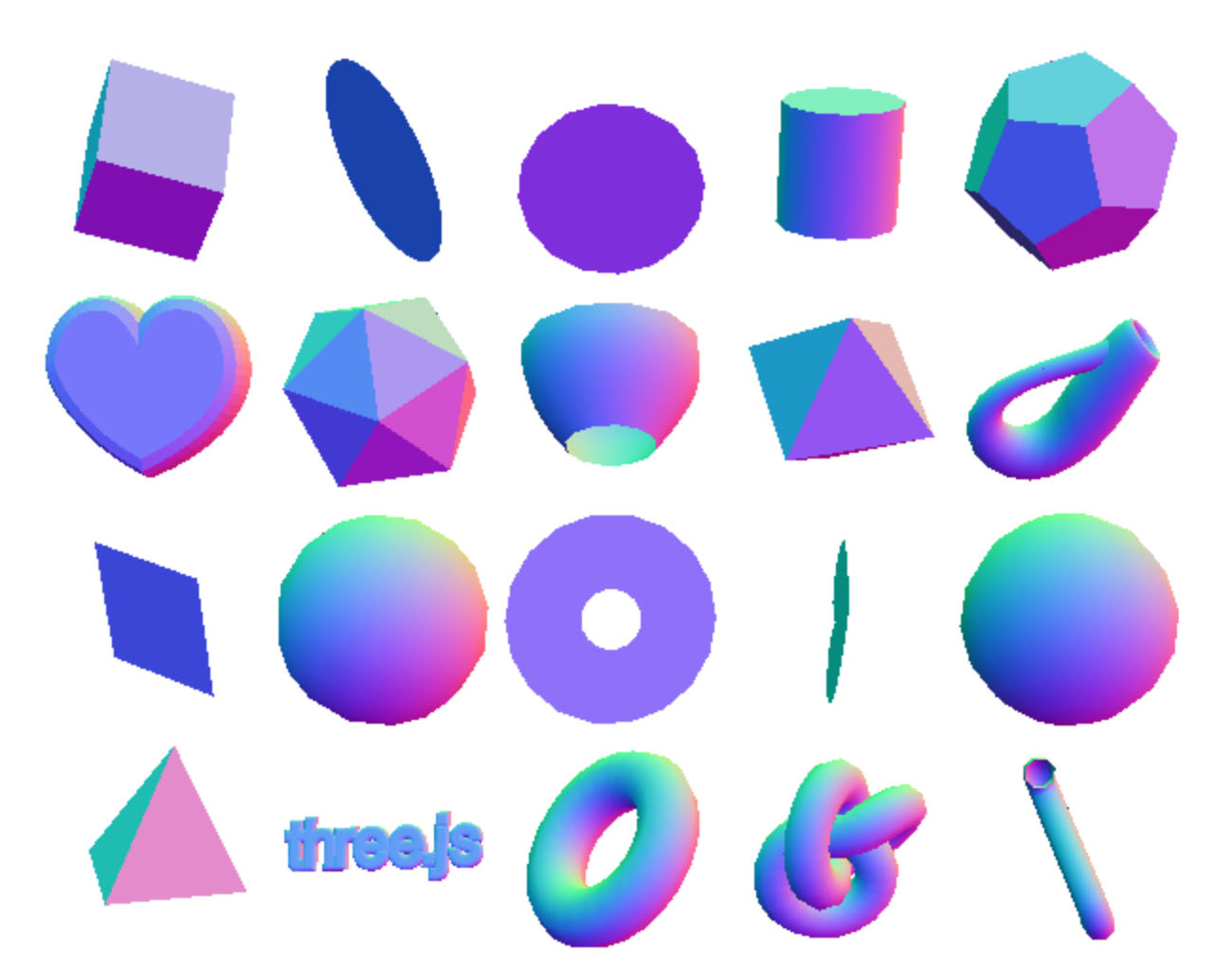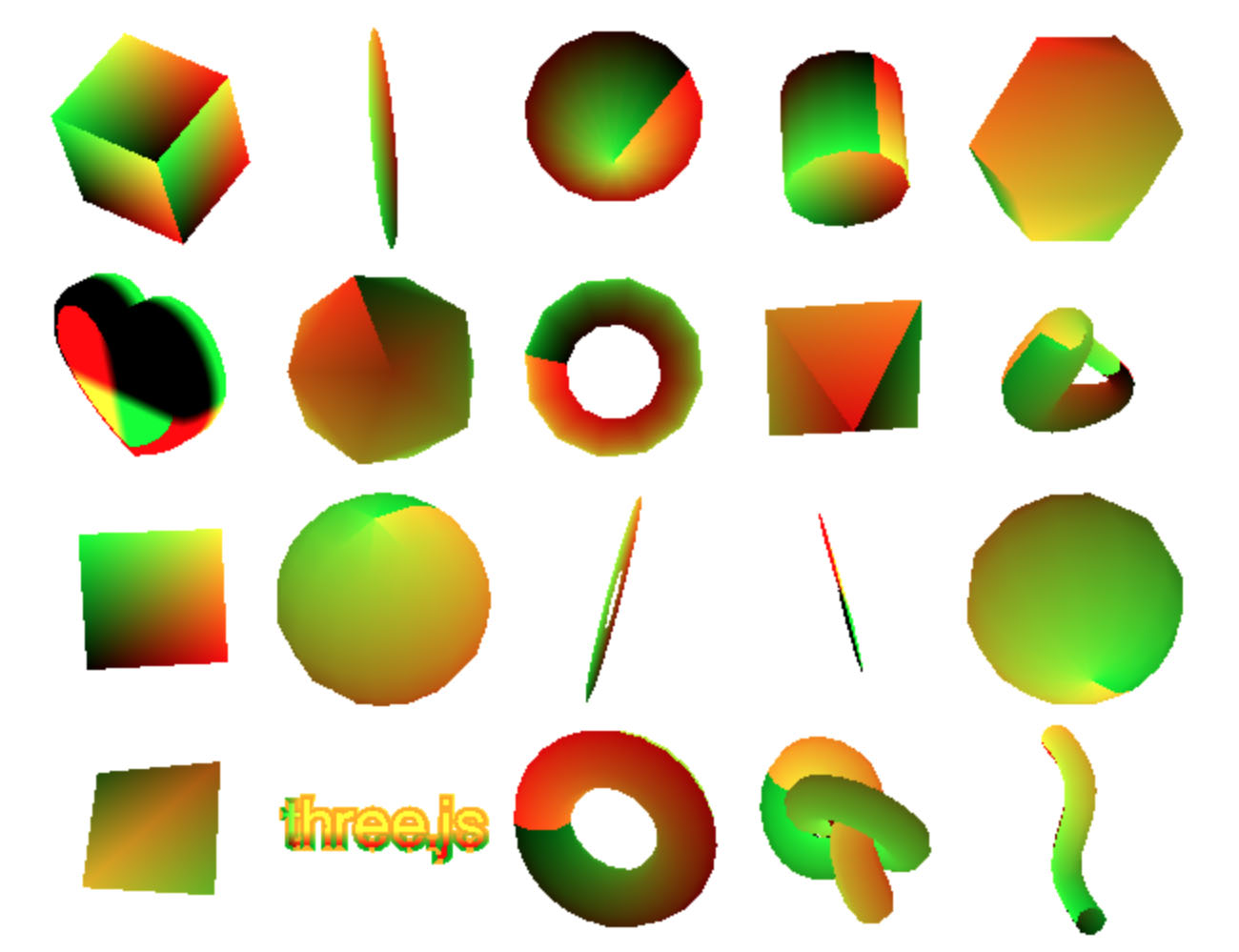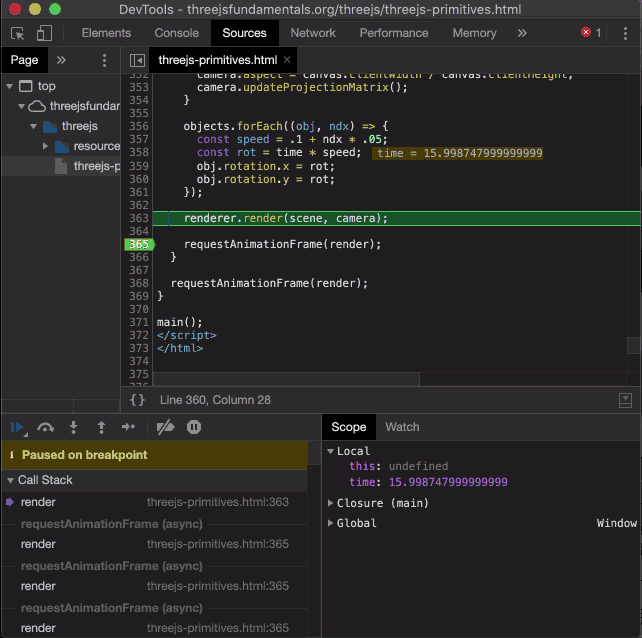# 在中调试GLSL

```void main() {

...

gl_FragColor = vec4(1, 0, 0, 1);  // red
}
```

```gl_FragColor = vec4(vNormal * 0.5 + 0.5, 1);
``````gl_FragColor = vec4(fract(vUv), 0, 1);
``````gl_Position = projection * modelView * vec4(position.xyz, 1);
```